# Abstracts

A Rao-Blackwellized particle filter has been designed and its performance investigated in a simulated three-axis satellite testbed used for evaluating on-board attitude estimation and control algorithms. Vector measurements have been used to estimate attitude and angular rate and, additionally, a pseudo-measurement based on a low-pass filtered time-derivative of the vector measurements has been proposed to improve the filter performance. Conventional extended and unscented Kalman filters, and standard particle filtering have been compared with the proposed approach to gauge its performance regarding attitude and angular rate estimation accuracy, computational workload, convergence rate under uncertain initial conditions, and sensitivity to disturbances. Though a myriad of filters have been proposed in the past to tackle the problem of spacecraft attitude and angular rate estimation with vector observations, to the best knowledge of the authors the present Rao-Blackwellized particle filter is a novel approach that significantly reduces the computational load, provides an attractive convergence rate, and successfully preserves the performance of the standard particle filter when subjected to disturbances.

Nonlinear filtering; Rao-Blackwellized particle filter; extended Kalman filter; unscented Kalman filter; nonlinear dynamics

Filtragem não linear; filtro de partículas Rao-Blackwell; filtro de Kalman estendido; filtro de Kalman unscented; dinâmica não-linear

PROCESSAMENTO DIGITAL DE SINAIS

Rao-Blackwellized particle filter with vector observations for satellite three-axis attitude estimation and control in a simulated testbed

Filtro de partículas Rao-Blackwell com medidas vetoriais para estimação e controle em 3 eixos da atitude de satélite testado em simulação de plataforma posicionadora

Ronan Arraes Jardim Chagas; Jacques Waldmann

Dept. System and Control, Division of Electronics Engineering Instituto Tecnológico de Aeronáutica CEP 12228-900 - São José dos Campos, SP, Brazil. ronan.jardim@gmail.com, jacques@ita.br

ABSTRACT

A Rao-Blackwellized particle filter has been designed and its performance investigated in a simulated three-axis satellite testbed used for evaluating on-board attitude estimation and control algorithms. Vector measurements have been used to estimate attitude and angular rate and, additionally, a pseudo-measurement based on a low-pass filtered time-derivative of the vector measurements has been proposed to improve the filter performance. Conventional extended and unscented Kalman filters, and standard particle filtering have been compared with the proposed approach to gauge its performance regarding attitude and angular rate estimation accuracy, computational workload, convergence rate under uncertain initial conditions, and sensitivity to disturbances. Though a myriad of filters have been proposed in the past to tackle the problem of spacecraft attitude and angular rate estimation with vector observations, to the best knowledge of the authors the present Rao-Blackwellized particle filter is a novel approach that significantly reduces the computational load, provides an attractive convergence rate, and successfully preserves the performance of the standard particle filter when subjected to disturbances.

Keywords: Nonlinear filtering, Rao-Blackwellized particle filter, extended Kalman filter, unscented Kalman filter, nonlinear dynamics.

RESUMO

Palavras-chave: Filtragem não linear, filtro de partículas Rao-Blackwell, filtro de Kalman estendido, filtro de Kalman unscented, dinâmica não-linear.

1 INTRODUCTION

Nonlinear estimation has been investigated here based on extended Kalman, unscented, and particle filtering to gauge the performance tradeoff among attitude and angular rate estimation accuracy, robustness to uncertain initial conditions and model mismatch, and computational workload. This investigation has been motivated by an experimental setup in the LabSim at the Instituto Nacional de Pesquisas Espaciais (INPE), where a 3-axis, air-suspended table has been instrumented as a testbed for designing and testing satellite attitude estimation and control algorithms, and systems integration. The apparatus has been used as an educational tool in demonstrations to undergraduate and graduate students from ITA (Instituto Tecnológico de Aeronáutica), and stirred up such an interest that inspired the present effort to simulate a model of a similar testbed for the evaluation of nonlinear estimation algorithms and their feasibility for actual space applications, specifically the attitude control system of a low-cost university satellite.

Recent published work on applying particle filtering, extended and unscented Kalman filtering (Chagas and Waldmann, 2010; Chagas and Waldmann, 2010a) to the aforementioned simulated satellite testbed has shown that the sequential Monte Carlo method performs significantly better in case an unexpected disturbance occurs and yields faster convergence. The state vector spans a 7-dimension space, and thus a high number of particles is needed to achieve a good approximation to the minimum mean square error (MMSE) estimate (Ristic et al., 2004). Hence, the heavy computational burden of the particle filter prevents a real-time implementation even in desktop PCs (Chagas and Waldmann, 2010).

Crassidis and Markley (2003) have proposed an unscented Kalman filter (UKF) with rate-gyros and magnetometer measurements as an alternative to the use of the standard extended Kalman filtering (EKF) approach to a similar problem of spacecraft attitude and angular rate estimation, and verified improved convergence with respect to the EKF. Cheng and Crassidis (2009), using the same measurements, have proposed a particle filter (PF) with a technique called progressive correction and verified that the initial convergence rate was much better than EKF and UKF for large initialization errors. Finally, Carmi and Oshman (2009) have proposed a fast PF with a novel initialization and some additional steps that help to reduce drastically the number of particles needed with respect to the conventional, plain PF, also called bootstrap filter.

The previous investigations mentioned above have analyzed the respective algorithms from the point of view of initial convergence rate, and the conclusions were that the particle filter yields a faster convergence rate than EKF and UKF. However, to the best knowledge of the authors, filter performance under high disturbance torques has not been investigated because its occurrence is very unlikely in actual space applications. But the satellite testbed on the ground may need to deal with this kind of disturbance, and it turned out that in such a circumstance conventional PF algorithms with a low number of particles can cause estimation divergence (Chagas and Waldmann, 2010). One way to circumvent this problem is to reinitialize the particles once the disturbance has been detected. However, a much less computationally demanding approach based on the Rao-Blackwellization technique (Akashi and Kumamoto, 1977) with a fairly small number of particles is proposed here that shows a disturbance rejection comparable to that of the conventional, plain PF with a large number of particles.

The present investigation extends previous work on PF for the simulated satellite testbed (Chagas and Waldmann, 2010a; Chagas and Waldmann, 2010) but now with the main focus on a Rao-Blackwellized PF. The procedure reduces the variance of Monte Carlo estimates and is applicable when, conditioned on a set of states, the remaining ones are linear and Gaussian (Doucet, 1998). Liu et al. (2007) have investigated a similar approach known as the marginalized PF applied to attitude and rate-gyro bias estimation with vector observations and also resorting to rate-gyro measurements. Here, the state vector has been partitioned into two groups: one with attitude-related components and the other with angular rate components. Samples have been taken from the second group with nonlinear dynamics, whereas the components in the first group, which are also nonlinear, are estimated using an Extended Kalman filter. Therefore, unlike the model studied at Liu et al. (2007), the system model here is not conditionally linear, but the Rao-Blackwellization approach becomes applicable by use of some mild approximations. Moreover, a significant reduction of the number of particles to attain an estimation accuracy much similar to that of the standard particle filter has been attained by concatenating pseudo-measurements of the angular rate to the measurement vector. The pseudo-measurements have resulted from low-pass filtering the numerical time-derivatives of the vector measurements. Though suboptimal, the present approach becomes extremely attractive due to its reduced computational workload, which yields the Rao-Blackwellized PF a potential algorithm for this space application.

In Brazil, most of the previous investigations on estimation and control of satellite attitude were carried out at INPE, such as Lopes et al. (1998), Walter and Pinto (1999), Silva et al. (2004), Conti et al. (2007), Castro et al. (2008), Louro et al. (2007), and Duarte et al. (2009). Optimal nonlinear filtering in GPS/INS integration was studied by Carvalho et al. (1997). Regarding nonlinear estimation of satellite attitude, Rios Neto et al. (1982) proposed an adaptive Kalman filter. Santos and Waldmann (2009) compared an extended and an unscented Kalman filter with vector observations from a Sun sensor and magnetometer for a low-cost satellite (ITASAT) attitude and angular velocity estimation. Garcia et al. (2011) developed and compared two different unscented Kalman filters that were based on quaternion and Euler angles for attitude parameterization.

The system model is presented in Section 2. The control strategy is briefly described in Section 3. Section 4 contains information about the filters that have been designed for this investigation. Finally, simulation results and conclusions are presented in Sections 5 and 6.

2 SYSTEM MODEL

The actual table mass unbalance and corresponding pendulous effect due to gravity torque has been neglected in the simulated testbed (Fig. 1). This inconvenience can be circumvented by careful balancing of the table mass prior to application of the results presented here. Sun sensors on board an orbiting satellite provide a reference direction for attitude estimation and control. This reference direction has been simulated in the simulated testbed by use of accelerometers measuring the local vertical given by the reaction to the gravity vector, and assuming that the horizontal acceleration was negligible. Hence, one requirement for the control system was that it should align the table with the local horizontal plane. Hence, the table has been instrumented with two accelerometers with mutually orthogonal sensitive axes parallel to the table surface. Additionally, one 3-axis magnetometer on board the table has been used to provide a measurement of the required additional reference direction, namely the local geomagnetic field, which has also been measured by an external, horizontally aligned, ground-fixed 3-axis magnetometer. The actuator suite is composed of a momentum wheel for azimuth control (Carrara and Milani, 2007) within 1° relative to a desired direction, and compressed air nozzles for on-off torquing the table towards alignment within 0.5° relative to the local horizontal plane. Notice that the focus is on nonlinear attitude and angular rate estimation. Therefore a conventional control strategy has been devised assuming linearized dynamics and pole placement with feedback of the state estimate. The system model has been already developed in Chagas and Waldmann (2010a), and is again presented here for the sake of completeness.

2.1 Coordinate Frames

Three coordinate frames have been used to derive an adequate model. The first one is the body-fixed coordinate frame {Xb, Yb, Zb}, which is attached to the table with the Zb axis perpendicular to the table plane and pointing upward. The second coordinate frame is the desired reference frame {Xd, Yd, Zd}, which is aligned with the external magnetometer sensitive axes. Non-orthogonality in the external magnetometer axes has been neglected. Both b and d frames are shown in Fig. 1. The rotation sequence has been parameterized by Euler angles ψ, θ, and Φ, respectively about body axes Zb, Yb, and Xb, thus rotating a vector representation from the desired reference frame to the body frame. Note that here the inertial coordinate frame neglects the Earth's rotation rate.

The desired reference frame has been useful for comparing the on-board magnetometer measurements with respect to the external magnetometer data. Additionally, a horizontal coordinate frame {Xh, Yh, Zh} results from rotating the body-fixed, table coordinate frame with the above Φ and -θ Euler angles about Xb, and Yb axes, respectively. The horizontal frame when rotated by angle y about the positive upward, local vertical yields the alignment with the desired reference frame. This is shown in Fig. 2.

2.2 Sensors

Attitude estimation relies on three sensors on board the air-suspended table: two accelerometers and one magnetometer. The accelerometers are used to estimate the local vertical and thus determine the misalignment between the table and the horizontal coordinate frame. Data from the on-board magnetometer, called M1, should be compared with the output of the external magnetometer, called M2, to determine the error with respect to the reference azimuth direction about the local vertical.

The two accelerometers measure the components of specific force along the Xb and Yb axes, respectively Aspb,1 and Aspb,2, due to the reaction to gravity in the body-fixed, table coordinate frame, as in Eq. 1:

where Ddb is the direction cosine matrix (DCM) that transforms a vector representation from the desired reference frame to the table coordinate frame. Accelerometer bias and measurement noise have not been considered in Eq. 1, but were taken into account when validating and comparing the performance of the closed-loop control law with feedback of state estimates computed by the distinct estimators.

Both magnetometers have been assumed to be located such that the local magnetic field vector is practically the same at both locations. Otherwise, comparing their respective measurements would not be useful for estimating the desired reference azimuth, thus compromising accuracy when estimating Euler angle ψ.

The magnetometer on board the air-suspended table outputs a vector measurement, M1b, which calls for representation in the horizontal coordinate frame. The representation has been carried out with the estimated Euler anglesandto approximate the DCM, as in Eq. 2.

One can compare M1h and M2b and use the cross product operation to estimate sin (ψ), thus yielding Eq.3:

where Mxd,y is the y-th component in the d coordinate frame of the unit-norm geomagnetic field measurement vector produced by the x-th magnetometer. Therefore, the sensor suite described here allows for the measurement of the three Euler angles that rotate the desired reference coordinate frame into alignment with the air-suspended table frame.

2.3 Actuators

A set of three actuators has been considered to control the air-suspended table about its three axes: two pneumatic actuators for the Xb and Yb axes, and one reaction wheel forZb. The pneumatic actuators are assumed to be controlled by a pulse width modulation (PWM) signal that determines the duty cycle. Additive white noise has been included in the actuator model to account for the small turbulence at the nozzles when torquing the table. Three parameters are called for in such a model: the torque magnitude that is applied on the table by the nozzles when the actuator is on, the frequency of the PWM carrier, and the actuator noise variance. The reaction wheel has been modeled as in Sidi (1997) for the purpose of validating estimation and control algorithms. This ground-truth model has included wheel motor dynamics, current and voltage limits, viscous friction, back-emf, and the maximum angular rate limit. The usual dead-band found in such wheels when crossing zero speed has not been considered since it was assumed that the wheel is used for attitude control with a significant non-zero angular rate. Hence the actuator actually behaves as a biased momentum wheel, and such simplifying assumption does not affect the generality of the results. The corresponding block diagram can be seen in Fig. 3, where Iw is the wheel inertia, Im,3 is the table inertia about the Zb axis, Kmw, Kvw, Rmw and Bw are electromechanical wheel parameters, Tw is the commanded torque, and u3 is the actual torque.

The wheel angular rate with respect to the air-suspended table ωtac measured by an on-board tachometer is composed of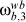and additive white Gaussian noise.

2.4 The Dynamics Model

The dynamics model has been adapted from Sidi (1997). Both the table inertia matrix without consideration of the reaction wheel Im,b, and the reaction wheel inertia matrix Im,b, are shown in Eqs. 4 represented in the body-fixed table coordinate frame b.

The table angular rate vector with respect to the inertial frame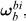and the reaction wheel angular rate vector with respect to the tableare shown in Eqs. 5 represented in the b coordinate frame:

Following the Newtonian formulation, the dynamics model is represented in the table coordinate frame b as in Eqs. 6:

with Td,b as the disturbance torque, Td,b being the control torque output by the pneumatic actuators and shown in Eq. 7, Hb is the total angular momentum of both the table and the reaction wheel as in Eq. 8, and u3 is the real torque acting on the reaction wheel as shown in Fig. 3. Mass unbalance torque due to gravity has been neglected because the testbed is balanced to align its center of mass with the table air bearing.

The table angular rate vector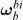relates to attitude Euler angles ψ, ψ, and Φ and respective time derivatives according to the kinematics in Eqs. 9:

Hence, the ground-truth model has been constructed using Eqs. 6, Eq. 8, and Eqs. 9, and the reaction wheel dynamics model seen in Fig. 3.

2.5 Model State and Measurement Vectors

Analyzing the model equations in the previous section, a state vector in R7 has been defined: the three Euler angles that rotate from the desired reference frame to the body-fixed table frame, the three components of the angular rate vector of the table with respect to the inertial frame represented in the body-fixed coordinate frame attached to the table, and the reaction wheel speed with respect to the table. Static friction torque in the reaction wheel yields a steady state pointing error about the Zb axis. Therefore, the integral of the pointing error shown in Eq. 10 has augmented the state vector as seen in Eq. 11:

The reference state is given by Eq. 12. Thus, the controller should align the table with the local horizontal plane, and likewise the on-board magnetometer measurements with those of the external magnetometer component-wise.

Recalling Eq. 1 and Eq. 3, the measurement vector concatenates accelerometers, magnetometers and tachometer data as in Eq. 13.

3 CONTROL STRATEGY

The main focus is to investigate and compare the performance of nonlinear estimators. Consequently, a straightforward control technique based on state feedback has been used. Firstly, the system has been linearized in the vicinity of xref. As a result, the horizontal plane dynamics given by the state components Φ, θ, and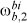has become decoupled from the vertical dynamics embedded in the remaining state components. Such decoupling allowed for the design of two separate control laws for the horizontal and vertical dynamics, respectively. Then, the closed-loop poles in Eqs. 14 have been located to avoid actuator saturation while still yielding an acceptable settling time.

Additionally, control torque about each horizontal axis is turned off when the corresponding Euler angle error norm is less than 0.25°. Control is switched back on when such error norm is higher than 0.5°. This avoids high-frequency switching in actuators when the system is near the reference state.

4 ESTIMATORS

This section describes the implementation of the estimators, which assumed model simplifications. Disturbance torques have been neglected, inertia matrices considered diagonal, i.e., without inertia products, and the reaction wheel friction and back-emf have been neglected, i.e. Tw = u3.

The continuous mathematical model, omitting model and measurement noise, can be written as in Eqs. 15:

where u is a vector of control torques for both of the pneumatic actuators and the reaction wheel, and f(.) is a function concatenating Eqs. 4 to Eq. 10 while considering the aforementioned simplifications.

The PF algorithms call fora discrete-time system model. The discretization is carried out, omitting model and measurement noise, as in Eqs. 16:

where Δ denotes the sample time. Function fΔ(xk-1 ,uk-1), which transfers the system from instant k - 1 to instant k using the sample time Δ, can not be computed analytically due to the nonlinear behavior of the continuous dynamics. Therefore it has been approximated using the Runge-Kutta 4th-order algorithm with a fixed time step.

The ground-truth model has not considered any approximation; it has been built using Newton's laws and as such does not involve any modeling noise. However, implementation artifacts contribute to a mismatch between the ground-truth model and the one embedded in the estimators. These errors, including those arising from numerical round-off, have been merged into a virtual, additive, white noise sequence approximated by a Gaussian probability density with zero mean and a covariance that should be tuned in the estimator.

4.1 Extended Kalman Filter (EKF)

Regarding Eqs. 16 augmented with the corresponding additive noise, the EKF performs the linearization of the dynamics about the updated state estimate, whereas the linearization of the measurement equation is about the propagated state estimate (Ristic et al., 2004). The continuous-discrete approach for the EKF has been used here (Gelb, 2001). Being a slightly different algorithm with respect to the usual fully discrete-time implementation, the approach offers more adequate, improved performance in continuous systems than the fully discrete approach because propagation is done by directly integrating the nonlinear continuous-time model equations as in the continuous Kalman-Bucy filter using, for example, the 4th-order Runge-Kutta numerical integration algorithm with a fixed time step, whereas the measurements are discrete in time. Propagation is shown in Eqs. 17:

where Jf(x, u) is the Jacobian matrix of the function f(.) at the updated estimate and control pair (x,u), and Q is the continuous-time model noise power spectrum density matrix. The update step is performed as usual, and the above EKF has been implemented previously in Chagas and Waldmann (2010a).

4.2 Unscented Kalman Filter (UKF)

The UKF uses the unscented transform to achieve improved estimation accuracy relative to the EKF when Eq. 15 augmented with additive noise is a highly nonlinear model of system dynamics and measurements. The unscented transform calculates a set of σ-points that are propagated using the nonlinear model and measurement equations to yield estimates of the mean and covariance of the stochastic state vector (Ristic et al., 2004). Such estimates better approximate the linear minimum mean square error estimate in comparison with the EKF estimation. Unlike the latter, it does not call for computation of Jacobians. Nevertheless, computing σ-points requires a great amount of computational effort, which yields a heavier computational burden than the EKF in almost every practical situation. The UKF has been also implemented in Chagas and Waldmann (2010a) with the continuous-discrete approach described in Särkkä (2007).

4.3 Rao-Blackwellized Particle Filter (RBPF)

Particle filters can be inefficient when dealing with high dimensional systems because a large number of particles is needed to represent the posterior probability density (Murphy and Russell, 2001). Rao-Blackwellization is a technique to exploit the state-space structure and thus reduce the number of particles. It is well-suited when the system dynamics is conditionally Gaussian. That is, when conditioned on some of the state components, the remaining ones are linear and Gaussian so that the latter can be analytically estimated by the Kalman filter (Doucet, 1998).

Chagas and Waldmann (2010) verified that standard PF algorithms impose a huge computational burden because they sample from the entire state space to solve this problem, thus becoming unfeasible in actual space applications with the presently available computational resources. Unfortunately, the satellite simulation testbed model can be conditionally Gaussian only when conditioned on a high-dimensional sub-space, which does not decrease the computational burden significantly. However, it turns out that the RBPF can be successfully used with a significant improvement in performance when some approximations are made.

Firstly, the integral of the azimuth pointing error has been removed from the state vector, and the integration has been performed for the purpose of control implementation with a simple rectangle rule and the azimuth estimate. So the state-space has been divided into two subspaces spanned, respectively, by r and z as presented in Eqs. 18.

Chagas and Waldmann (2010) verified that the standard PF with a regularization step does not provide accurate estimation of angular rate even when a large number of particles are used. The measurement equation in Eq. 13 shows that the subspace spanned by the body angular rates is not directly measured and a large number of particles would be needed to sample that subspace and achieve the required estimation accuracy. The need for sampling the importance density with many particles is caused by the angular rate being absent from the measurement vector. Consequently, information is not available in the measurements to guide the sampling towards the subspace regions with high likelihood and obviate the need for many particles. As a result, many particles are called for, and sampling is performed based just on the propagation of the dynamics model embedded in the filter. Poor performance can occur in case disturbances occur though. Thus, a pseudo-measurement of angular rate has been used as a means of reducing the required number of sampling particles while maintaining estimation accuracy. This angular rate pseudo-measurement has been produced by means of differentiating and low-pass filtering the measurement equation, and then particle samples of the angular rate with a high likelihood have been generated. A method to achieve this goal has been inspired by previous work on angular rate estimation from vector observations (Bar-Itzhack, 2001) and is used here with a slight modification as described next.

Taking the time derivative of the first, second and third components of the measurement vector, recalling the attitude kinematics in Eq. 9, and from Eq. 1, Eq. 3, and Eq. 13 one obtains Eqs. 19 (see proof in Appendix AAppendix A):

These derivatives can be approximated as in Eq. 20:

where yi,k is the i-th component of the original measurement vectorinEq. 13 at instant k. Bar-Itzhack (2001) has proposed the use of a pseudo-inverse function to estimate the angular rate vector from yP,k. Here, it is the inverse of D(Φ, θ, ψ), which always exists when the Euler angles are subject to -90° < Φ, θ, ψ < 90°. One should note that estimation of the Euler angles is needed for pseudo-measurement computation, and hence the most recent r subspace estimates have been employed for that purpose. Finally, the angular rate vector estimation has been obtained as in Eq. 21:

whereis the updated estimate in the r subspace at instant k  1. Finally, the pseudo-measurement vector in Eq. 20 is low-pass filtered to attenuate noise arising from the numerical time-derivatives (Franklin et al., 1997) as in Eq. 22:

where Δ is again the sample time and τ is the filter time constant. Finally, the augmented measurement vector has been partitioned as in Eq. 23:

where Hz is the 4 x 4 identity matrix and the measurement function hr(rk) can be easily constructed from Eq. 1 and Eq. 3. Notice that the measurement equation of the z sub-space actually has a dependence on the r subspace because of the coupling with attitude seen in Eq. 21. However, the correct mathematical treatment for this dependence in the PF algorithm would raise filter complexity to an undesirable level. As a simplifying assumption, such coupling will be neglected here.

With the past results, the discrete model and measurements equations can be written as shown in Eqs. 24.

where, wz,k ~ N(0, Qz) , wr,k ~N(0, Qr), vz,k ~ N(0, Rz), vr,k ~ N(0, Rr) and all these random vectors are considered to be white and mutually independent. One can see that fz(zk1, uk1) is obtained from discretization of Eqs. 6, and fr (r1, zk1)from discretization of Eqs. 9 with the Runge-Kutta algorithm, as mentioned earlier in Eqs. 16.

Applying the PF algorithm with particle samples taken just from zk, the minimum mean square error (MMSE) estimate can be approximated as in Eqs. 25 (Doucet, 1998):

where zk(j) is the j-th particle in the z subspace, wjk is the associated weight, and N is the total number of particles.

Notice that the expectationcannot be analytically computed because of the nonlinear model of the dynamics embedded in the r subspace, but has been approximated by an EKF. This approach is widely used and corresponds to approximate the probability density function (P.D.F.)by a Gaussian distribution with parameters calculated by the EKF (Giremus and Tourneret, 2005). Hence, a set with N EKFs has been constructed, with each EKF using functionas the model of the r subspace dynamics.

The r subspace has been selected for estimation with the sets of EKFs due to the dynamics model in Eqs. 24. One can see that the z subspace dynamics does not depend on the r sub-space. Hence, sampling the z subspace depends on just the particles set at the past instant and on the measurement at the present instant. On the other hand, sampling the r subspace would need the particles set at the past instant, the measurement at the present instant, and the z subspace state components at the past instant. The mathematical treatment in such a case would increase the complexity of the estimation algorithm. Hence, the estimation is carried out by a Monte Carlo-based method in the z subspace, and by a Kalman filter-based method for the remaining state components in the r subspace (Doucet, 1998).

The importance density has been chosen as the optimal one that minimizes the variance of the weights and is useful to reduce the total number of particles (Doucet, 1998):Sampling from this P.D.F. can be easily done using theorem 1.

Proof: See Appendix BAppendix B. □

One can see from Eq. 23 that the measurement yz,k on which the importance densityis conditioned linearly relates to the state partition zk, and the measurement noise is assumed additive and Gaussian. It can be proven that this P.D.F is the one presented in Eq. 26 (Doucet, 1998):

where N(x; m, R) is the Gaussian probability density with mean m and covariance R evaluated at x.

The particle weights update is carried out as stated in theorem 2.

Theorem 2 The particle weights update step can be approximated by Eq. 27:

with Ck-1being a normalization constant that does not depend onthe predicted estimate for the k-th instant of the j-th EKF using all measurements up to the k - 1 instant with covariance matrix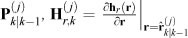, and.

Proof: See Appendix CAppendix C. □

The standard, plain PF (i.e. the bootstrap filter) algorithm performance degrades when the dynamics model noise level is low, because after a few time steps all particles tend to concentrate in a very tight region in the state-space (Ristic et al., 2004). Here, all modeling noise is caused mainly by model mismatch and numerical errors. Such noise has a very small covariance and the standard PF performance degrades significantly. To avoid such a hurdle, a movement step has been added after every resample stage using the Metropolis-Hastings algorithm (Chilb and Greenbeg, 1995; Ristic et al., 2004). Finally, the full algorithm can be described:

Initialization:

• FOR

j = 1 :

N DO

-z0(j) ~ p0(z), where p0(z) is the prior probability of the z subspace at instant 0.

-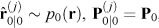, where p0(z) is the prior probability of the r subspace at instant 0 and P0 is the initial EKF filter covariance. Notice that the prior probability does not need to be Gaussian. It can be, for example, an uniform distribution that covers the entire r subspace, but P0 must be set accordingly to avoid divergence.

• END FOR

At every instant k:

• Construct the pseudo-measurement ŵ

f,k using Eq. 20, Eq. 21, and Eq. 22, and assemble the measurement vector

y

z,k of the

z subspace as in Eqs. 23.

• FOR

j = 1 :

N DO

- Draw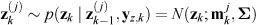, where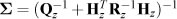and.

- Useto execute the prediction step of the j-th EKF to obtainand.

- Use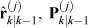and yr,k to execute the update step of the j-th EKF to obtain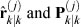.

- Update the j-th particle weight using:

• END FOR

•= NORMALIZE

• Compute

Neff

=

• IF

Neff <

Nthres

- Execute the resample step with systematic re-sample (Ristic et al., 2004) using the normalized weights to achieve a new setofparticlesand respective EKFs- Execute the movement step using the Metropolis-Hastings algorithm (Ristic et al., 2004) to achieve a new set of particles. Notice that the EKFs remain untouched, because they only depend on particles, whichremainthe same inthe movement step.

• END IF

•, which is the approximation of the minimum mean square error estimate for the

r subspace as in Eqs. 25 (Murphy and Russell, 2001).

•, which is the approximation of the minimum mean square error estimate for the

z subspace as in Eqs. 25 (Murphy and Russell, 2001).

4.4 Plain Particle Filter (PPF)

A standard, plain PF (PPF), which samples from the entire state space, has also been designed just to gauge the performance of the proposed RBPF. The standard PF has been implemented using systematic resample (Ristic et al., 2004) composed with a regularization step to increase the diversity of the particles (Ristic et al., 2004). More details about this particular implementation can be found in Chagas and Waldmann (2010).

5 SIMULATIONS AND RESULTS

5.1 Parameters

The simulations have been carried out using simulation table parameters available from (Carrara and Milani, 2007), and the XSens MTiG inertial measurement unit (IMU) specification sheet as shown in Table 1. Both table and reaction wheel ground-truth inertia matrices have included inertia products to account for a residual mass unbalance in the table assembly and uncertainty regarding the reaction wheel inertia parameters. Equation 28 shows the measurement noise covariance matrix used in EKF, UKF and PPF:

where diag(.) means a diagonal matrix. For the RBPF, the measurement covariance matrix has been partitioned according to Eqs. 24, and the used values are shown in Eqs. 29, with the appropriate SI units.

Each filter needed tuning of its model noise covariance as shown in Eqs. 30, with the appropriate SI units:

The number of particles in the PPF has been set to 500,000, and the RBPF has been simulated in distinct instances with 15, 500, and 5,000 particles.

5.2 Filter Performance

Two metrics have been defined to gauge filter performance. The first, the angle error, computes the rotation angle error about the Euler axis that is related to the attitude estimation error at each iteration as in Eq. 31. It has been used to ascertain the attitude estimation accuracy of each filter. The second, the angular rate error, computes the norm of the angular rate vector estimation error at each iteration as in Eq. 32.

where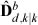and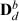are the estimated and ground-truth DCM, respectively, at instant k that rotate from the body-fixed coordinate frame attached to the simulation table to the reference coordinate frame, and ŵk|k and wk are the estimated and ground-truth table angular rate vector, respectively, at instant k. These two metrics have been computed at each iteration over a large number M of Monte Carlo simulations, and finally were employed to obtain the mean and standard deviation.

5.3 Results

All simulations have been carried out using Matlab in a controlled Linux environment (ArchLinux distribution with 2.6.36 kernel) to ensure that the relative computational burden of each algorithm can be properly estimated. For the EKF, UKF and RBPF scenarios, 100 Monte Carlo simulations have been carried out spanning a time interval from 0s to 100s. For the PPF, only 15 Monte Carlo simulations have been performed because of the heavy computational burden and the available time. The ground-truth initial state vector has been kept fixed and is given in Eq. 33 with SI units. For the Kalman filter-based simulations, the filter initial estimate has been set equal to the initial state vector plus a random vector in which each component was a random Gaussian variable with zero mean and variance 0.1 (SI units). For the PF-based simulations, the initial value for each particle has been sampled from a uniform distribution, whose limits for the angles have been set from 45° to +45°, and  10°/s to + 10°/s for the angular rates.

An unexpected, deterministic pulse disturbance torque has been applied at time t = 45s with a 0.3s duration to investigate filter robustness and convergence rate. The disturbing torque vector is given in Eq. 34.

The proposed RBPF has been simulated with 15, 500, and 5,000 particles, and the corresponding figures of merit in

Revista Controle & Automação/Vol.23 no.3/Maio e Junho 2012 287

Eqs. 31-32 for each scenario have been plotted in Fig. 4. To improve the analysis, a zoom of the angular rate error norm in steady state has also been plotted in Fig. 5. The comparison between EKF, UKF, PPF, and the proposed RBPF can be found in Fig. 6 and Fig. 7. Due to lack of space, additional results regarding the EKF, UKF and PPF can be found in Chagas and Waldmann (2010a) and Chagas and Waldmann (2010). Finally, Table 2 summarizes the computational burden results for each scenario. Figure 4 and Fig. 5 show that increasing the number of particles from 500 to 5, 000 has not improved RBPF attitude estimation, but provided a slightly improved angular rate estimation in steady state. Figures 6 and 7 show that even with a very low number of particles, the RBPF achieved an attitude determination accuracy that is comparable to the PPF with a huge number of particles. Furthermore, the angular rate estimation accuracy with just 15 particles is statistically the same as the PPF, but Kalman-based methods perform angular rate estimation better than the RBPF and PPF algorithms. The convergence rates of the PF-based estimation methods PPF and RBPF were better than that of the Kalman filter-based algorithms, with PPF yielding a slightly faster convergence than RBPF.

6 CONCLUSIONS

A Rao-Blackwellized particle filter has been designed to estimate satellite attitude and angular rate composed with a straightforward, linearized state feedback law for attitude control in a simulated, air-suspended table. The table uses two pneumatic actuators for alignment with the local horizontal plane, and one reaction wheel for azimuth alignment. The sensors consist oftwo accelerometers to estimate the local gravity vector direction, and two magnetometers - one on board the table, and the other fixed to the reference coordinate frame to provide azimuth alignment about the local vertical. The RBPF implementation proposed here for the particular application partitions the state-space into two groups: one formed by the attitude Euler angles and another with the angular rates. Particle sampling has been carried out just from the angular-rate subspace, whereas the Euler angles were estimated by a set of Extended Kalman filters. For that purpose, approximations have been employed to decrease the computational burden and to render the problem analytically tractable. In such a case achieving the minimum mean square error estimate is not granted though when the number of particles tends to infinity. Additionally, a significant reduction in the number of sampling particles has been accomplished by augmenting the measurement vector with low-pass filtering of the time-derivative of the vector observation. This augmented pseudo-measurement has guided the sampling of the angular-rate subspace, thus significantly decreasing the number of particles needed while simultaneously maintaining estimation accuracy.

The proposed RBPF yields a superior cost-benefit ratio than the PPF, because the former provided an estimation accuracy akin to the latter, and yet called for a much alleviated computational workload. However, by keeping a low number of particles the RBPF angular rate estimation accuracy has resulted inferior to those of the EKF or UKF. Moreover, both RBPF and PPF have produced attitude estimation far more robust to a torque disturbance. Therefore, when dealing with space applications that resort to sensors that measure vector observations and demand accurate angular rate control composed with the sound rejection of a disturbance torque - all of that without incurring in a heavy computational burden -, then the results reported here indeed encourage the use of the EKF or UKF running in parallel with the proposed RBPF with a small number of sampling particles.

Comparing with previous work on related applications, the particle reduction using the RBPF proposed here for the intended application is truly outstanding. Carmi and Oshman (2009) studied the problem of spacecraft attitude and angular rate estimation using vector observations, which is very similar to the problem presented here, and stated that the plain particle filter would need at least 20,000 particles to provide an acceptable estimation accuracy. Then, they designed a different particle filter with modifications of the initial sampling and additional steps to decrease the number of particles needed for attaining adequate estimation accuracy to just 1,800. For the application investigated here, Chagas and Waldmann (2010) verified that this number ought to be much higher for the PPF to avoid divergence when a large torque disturbance is applied - indeed, such is a benchmark for gauging estimator performance that has not been considered so far in previous work to the best knowledge of the authors. Since the proposed RBPF with just 15 particles has achieved a comparable estimation accuracy with respect to that of the PPF on Section 4.4 when subject to a torque disturbance and in steady state, it should be clear that the proposed RBPF algorithm shows a superb gain in terms of alleviating the computational workload.

Since the computational load of the RBPF with 15 particles is only about 3 times higher than that of the usual EKF, and has a disturbance rejection very similar to the PPF, its implementation has been considered feasible for this space application with adequate computational resources. Angular rate estimation quality can be improved with an architecture in which one EKF runs in parallel with the RBPF to accurately provide, respectively, angular rate and attitude estimates. Even in this scenario with both filters, the computational load will still be lower than that of an UKF. Having the EKF and RBPF running in parallel will demand the generation of a normally distributed sequence though, a feature that is not required by the UKF. Finally, an elaborate analysis of filter convergence demands further investigation.

APPENDICES

Differentiating the first three measurement vector components with respect to time one gets Eq. A. 1:

Equations 9 describe the kinematics relating the time derivative of the Euler angles and the table angular rate vector with respect to the inertial frame. Equation 19 is proved by means of substituting the kinematics in the Eq. A.1 and writing in a compact matrix form.

Firstly, using Bayes rule and process model present at Eqs. 24, one can see that:

Also:

Applying the total probability theorem and using Eqs. B.1 and B.2:

Finally the theorem can be proved by applying Bayes rule to the importance density thus yielding:

For the optimal importance density, the weights are updated using (Doucet, 1998):

Firstly notice that, when conditioned on rk, yr,k is independent of yz,k. Hence:

Also, using Bayes rule and process model present at Eqs. 24:

Using the total probability theorem in Eq. C.1:

Applying Eq. C.2 in Eq. C.4, one gets:

Finally, using Eq. C.3 in Eq. C.5:

Doucet (1998) showed that:

Also, the P.D.Fis approximated by a Gaussian density with mean and covariance computed by the set of extended Kalman filters:

It can be easily seen that:

Using this formulation, the integral in Eq. C.6 cannot be analytically solved due to the nonlinear function hr (rk). To circumvent this problem, this function is linearized aboutas follows:

where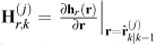. Hence, the P.D.F. expressed in

Eq. C.9 can be approximated by:

Therefore, using Eq. C.7, Eq. C.8, Eq. C.9, and Eq. C.11, the weights calculations in Eq. C.6 can be approximated by:

With this approximation, the aforementioned integral can be evaluated. Firstly, one should consider the Chapman-Kolmogorov equation applied to the prediction step of the standard Kalman filter, shown in Eq. C.13 (Arulampalam et al., 2002):

Both P.D.F. in the above integral are rewritten in the standard linear Kalman filter problem as follows (Ho and Lee, 1964; Anderson and Moore, 1979):

Performing the following variable substitution in Eq. C.14:

the vector dimensions remain consistent and the integral in Eq. C.12 can be calculated:

Finally, applying the result expressed in Eq. C.15 in Eq. C.12, one gets:

ACKNOWLEDGMENTS

The authors acknowledge the technical communications with INPE's researchers Dr. Hélio Koiti Kuga and Dr. Valdemir Carrara, and the support of project FINEP/DCTA/INPE Sistemas Inerciais para Aplicação Aeroespacial (SIA), embodied through the logistical support, acquisition of the computational resources needed for the research reported here, and the scholarship awarded to the first author.

Artigo submetido em 10/03/2011 (Id.: 1292)

Aceito sob recomendação do Editor Associado Prof. Ivan Nunes Da Silva

• Akashi, H. and Kumamoto, H. (1977). Random sampling approach to state estimation in switching environments, Automatica 13: 429-434.
• Anderson, B. D. O. and Moore, J. B. (1979). Optimal Filtering, Prentice-Hall, Englewood Cliffs, New Jersey, United States of America.
• Arulampalam, M. S., Maskell, S., Gordon, N. and Clapp, T. (2002). A tutorial on particle filters for online nonlinear/non-gaussian baysian tracking, IEEE Transactions on Signal Processing 50(2): 174-185.
• Bar-Itzhack, I. Y. (2001). Classification of algorithms for angular velocity estimation, Journal of Guidance, Control, and Dynamics 24(2): 214-218.
• Carmi, A. and Oshman, Y. (2009). Fast particle filtering for attitude and angular-rate estimation from vector observations, Journal of Guidance, Control, and Dynamics 32(1): 70-78.
• Carrara, V. and Milani, P. G. (2007). Controle de uma mesa de mancal a ar de um eixo equipada com giroscópio e roda de reação, V Simpósio Brasileiro de Engenharia Inercial, Rio de Janeiro, RJ, Brasil.
• Carvalho, H., Moral, P. D., Monin, A. and Salut, G. (1997). Optimal nonlinear filtering in gps/ins integration, IEEE Transactions on Aerospace and Electronic Systems 33(3): 835-850. Castro, J. C. V., Souza, L. C. G. and Kuga, H. K. (2008). Experimental angle and velocity estimation of a satellite simulator, V Congresso Nacional de Engenharia Mecânica, Salvador, BA, Brasil.
• Chagas, R. A. J. and Waldmann, J. (2010). Nonlinear filtering in a simulated three-axis satellite attitude estimation and control tested, Journal of Aerospace Engineering, Sciences and Applications 2(2): 37-49.
• Chagas, R. A. J. and Waldmann, J. (2010a). Nonlinear filtering in a simulated three-axis testbed for satellite attitude estimation and control, VI Congresso Nacional de Engenharia Mecânica, Campina Grande, PB, Brasil.
• Cheng, Y. and Crassidis, J. L. (2009). Particle filtering for attitude estimation using a minimal local-error representation, 2009 AIAA Guidance, Navigation and Control Conference, Chicago, IL, United States of America.
• Chilb, S. and Greenbeg, E. (1995). Understanding the metropolis-hastings algorithm, The American Statistician 49(4): 327-335.
• Conti, G. T., Souza, L. C. G. de. and Kuga, H. K. (2007). Control law design and parameters estimation of a satellite attitude control system simulator, 19th International Congress of Mechanical Enginering, Brasília, DF, Brasil.
• Crassidis, J. L. and Markley, F. L. (2003). Unscented filtering for spacecraft attitude estimation, Journal of Guidance, Control, and Dynamics 26(4): 536-542.
• Doucet, A. (1998). On sequential simulated-based methods for bayesian filtering, Technical Report CUED/F-INFENG/TR.310, Signal Processing Group, Department of Engineering, University of Cambridge, UK.
• Duarte, R. O., Filho, L. S. M. and Kuga, H. K. (2009). Performance comparison of attitude determination algorithms developed to run in a microprocessor environment, 20th International Congress of Mechanical Enginering, Gramado, RS, Brasil.
• Franklin, G. F., Powell, J. D. and Workman, M. L. (1997).Digital Control of Dynamics Systems, Addison Wesley Longman, Inc., California, Unites States of America.
• Garcia, R. V., Kuga, H. K. and Zanardi, M. C. F. de. P. S. (2011). Unscented kalman filter for spacecraft attitude estimation using quaternions and euler angles, 22nd International Symposium on Space Flight Dynamics, São José dos Campos, SP, Brasil.
• Gelb, A. (ed.) (2001). Applied Optimal Estimation, MIT Press, United States of America.
• Giremus, A. and Tourneret, J. (2005). Joint detection/estimation of multipath effects for the global positioning system, 30th IEEE International Conference on Acoustic, Speech, and Signal Processing, Philadelphia, PA, United States of America.
• Ho, Y. C. and Lee, R. C. K. (1964). A bayesian approach to problems in stochastic estimation and control, IEEE Transactions on Automatic Control 9: 333-339.
• Liu, Y., Jiang, X. and Ma, G. (2007). Marginalized particle filter for spacecraft attitude estimation from vector measurements, Journal of Control Theory and Applications 5(1): 60-66.
• Lopes, R. V. F., Carvalho, G. B. and Silva, A. R. (1998). Star identification for three-axis attitude estimation of french-brazilian scientific micro-satellite, Advances in the Astronautical Sciences 100(2): 805-819.
• Louro, A. C., Lopes, R. V. da. F. and Kuga, H. K. (2007). Fault diagnose in the autonomy micro-satellite attitude determination using gps and gyros, 19th International Congress of Mechanical Enginering, Brasília, DF, Brasil.
• Murphy, K. and Russell, S. (2001). Rao-Blackwellized particle filtering for dynamic bayesian networks, in A. Doucet, N. de Freitas and N. Gordon (eds), Sequential Monte Carlo Methods in Practice, Springer Verlag, New York, United States of America.
• Rios Neto, A., Lopes, R. V. F. and Paiva, R. N. (1982). Estimação adaptativa de atitude de satélites artificiais via filtro de kalman com compensação do modelo dinâmico, IV Congresso Brasileiro de Automática, Campinas, SP, Brasil.
• Ristic, B., Arulampalam, S. and Gordon, N. (2004). Beyond the Kalman Filter: Particle Filters for Tracking Applications, Artech House, Boston, United States of America.
• Santos, D. A. and Waldmann, J. (2009). Attitude and angular rate estimation from vector measurements of magnetometer and sun sensor for the low-cost itasat satellite,20th International Congress of Mechanical Enginering, Gramado, RS, Brasil.
• Sidi, M. J. (1997). Spacecraft Dynamics and Control: A Practical Engineering Approach, Cambridge University Press, New York, United States of America.
• Silva, C. M. de. L., Kuga, H. K. and Lopes, R. V. da. F. (2004). Estimação conjunta de atitude e órbita de satélites artificiais estabilizados por rotação utilizando observações do magnetômetro e sensor solar, Terceiro Congresso Temático de Dinâmica e Controle, Ilha Solteira, SP, Brasil.
• Särkkä, S. (2007). On unscented kalman filtering for state estimation of continuous-time nonlinear systems, IEEE Transactions on Automatic Con trol 52(9): 1631-1641.
• Walter, F. and Pinto, L. H. M. (1999). A new gps-based attitude estimation technique for spinning satellites, 26th URSI General Assembly, Toronto, ON, Canada.

# Appendix A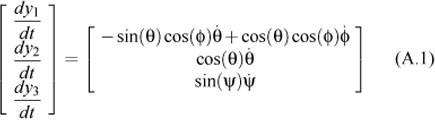# Appendix B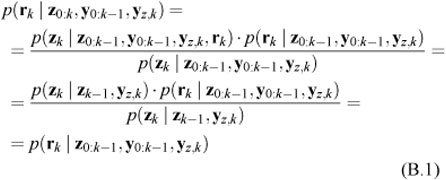# Appendix C# Publication Dates

• Publication in this collection
21 June 2012
• Date of issue
June 2012